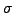Next: *ELECTROMAGNETICS Up: Input deck format Previous: *ELASTIC   Contents

## *ELECTRICAL CONDUCTIVITY

Keyword type: model definition, material

This option is used to define the electrical conductivity of a material. There are no parameters. The material is supposed to be isotropic. The constant may be temperature dependent. The unit of the electrical conductivity coefficient is one divided by the unit of resistivity (in SI-units: ohm) times the unit of length.

First line:

• *ELECTRICAL CONDUCTIVITY

Following line:

•.
• Temperature.
Repeat this line if needed to define complete temperature dependence.

Example:

*ELECTRICAL CONDUCTIVITY
5.96E7


tells you that the electrical conductivity coefficient is 5.96E7, independent of temperature (if SI-units are used this is the electrical conductivity of copper).

Example files: induction.

guido dhondt 2018-12-15